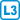## Point coloring

A set with $$(q + 1)^2$$ points is given. Each point $$u$$ is assigned a set of colors $$L (u)$$ of size $$q + 1$$. In addition, for any two different points $$u$$, $$v$$ applies $$| L (u) \cap L (v) | \leq 1$$.
Prove that points can be colored so that each point $$u$$ gets a color from $$L (u)$$ and different points are colored with different colors.